# Solution assignment 08 Fractional functions and graphs

### Assignment 8

Given the function: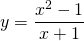Find:
the vertical asymptote;
the horizontal asymptote;
the intersection point with the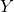-as if it exists;
the intersection point with the-as if it exists.

Based on these results sketch the result in the figure.

### Solution

The function looks like a fraction but actually it is not. We can simplify the function: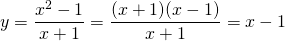voorActually the function is a straight line which is not defined for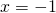. However, we can see:The graph of the original function is actually a straight line with a small 'hole' for. At the same time we know that the function approaches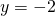ifapproaches (either from the left or the right)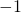.

0